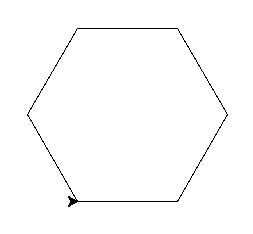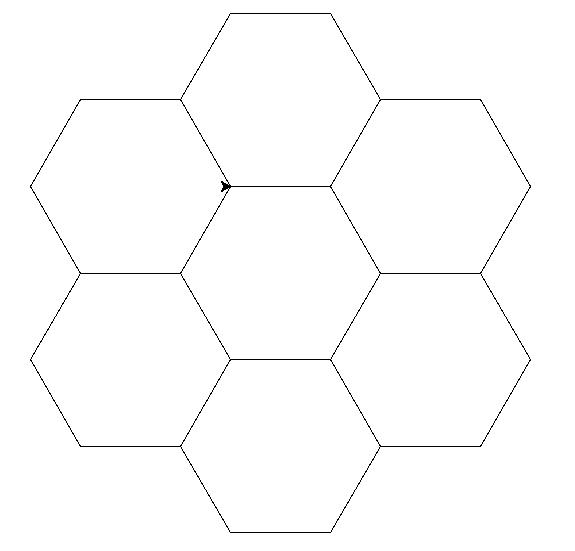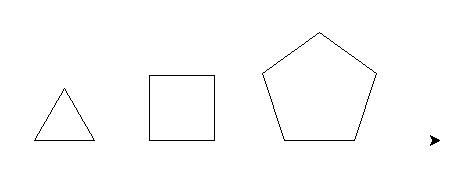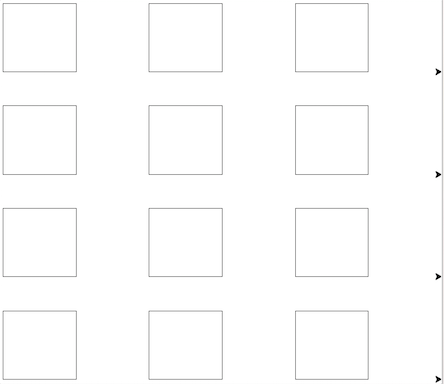# Data Structures¶

Data structures contain other objects. We will look at two: lists, dictionaries.

Typical methods defined on data structures are ones that allow access and update items within it.

As always first we explore how to create objects using literals and constructors, we then examine some methods typical of each object.

Second, we often want to do something for each item in a data structure. This involves ‘iterating’ over it. We do this using the for loop.

## Lists¶

A list object contains ordered items.

### Creation¶

```>>> ['John', 'Eric', 'Michael', 'Terry']          # literal
>>> list('abc')                                   # `list` transform a string
['a', 'b', 'c']
>>> type([1, 2, 3])
<class 'list'>
```

### Extraction & Update¶

```>>> abc = ['a', 'b', 'c']
>>> abc                        # extract item
'a'
>>> abc = 'd'                  # update item
>>> abc
['a', 'b', 'd']
```

Tip

Lists are 0 indexed. The first item is at index 0, the second at 1...

If you ask for an item that is outside of the list’s length you will get an IndexError.

### range¶

The range function combined with the list constructor is a fast way to create a list with a specified number of increasing integers.

```>>> list(range(3))   # think: give me numbers up to 3
[0, 1, 2]
```

It provides a convenient shortcut to do things a certain number of times.

```>>> for i in range(2):
...    print('hi')
'hi'
'hi'
```

## Dictionaries¶

Dictionaries contain key value mappings.

They can be used to collect information (data) about something. Here we use a dictinary to represent a Person.

### creation¶

```>>> {'name': 'Brian', 'age': 23, 'sex': 'M'}              # literal
>>> dict([('name', 'Brian'), ('age', 23), ('sex', 'M')])  # constructor
```

### extraction & update¶

Special syntax: <dict-name>[<key>] for extracting and updating an attribute.

```>>> person = {'name': 'Brian', 'age': 23, 'sex': 'M'}
>>> person['name']                          # extract value
'Brian'
>>> person['name'] = 'Naomi'                # update value
>>> person['name']
'Name'
```

If you request a non-existent key you get a KeyError.

## Nesting¶

Data structures can include any type of object including other data structures.

Here is a list of dictionaries:

```>>> persons = [
{'name': 'Naomi', 'age': 32, 'sex': 'F', 'status': 'Single'},
{'name': 'Jane', 'age': 29, 'sex': 'F', 'status': 'Married'},
{'name': 'Brian', 'age': 23, 'sex': 'M', 'status': 'Single'}
]
```

Nested data structures are extremely common.

Think how this could be useful for example to store information about all students in a class.

## The for loop¶

Use for to iterate over each item in a given list.

Here by iterate through a list of str objects we change the colour of our turtle alex.

```from turtle import Turtle, exitonclick

alex = Turtle()

for a_colour in ["yellow", "red", "purple", "blue"]:
alex.color(a_colour)
alex.forward(50)
alex.left(90)

exitonclick()
```

### Refactoring square¶

We refactor square combining range with a for loop.

```def square(side):
for i in range(4):
turtle.forward(side)
turtle.left(90)
```

Drawing a square is reduced to repeating the same action four times.

Thanks to the for loop our definition of a square in code:

• is shorter and more readable.
• communicates an insight into the geometry of a square.

## Exercises¶

### Refactor shapes.py¶

Refactor all the shapes in shapes.py and make good use of loops where you can.

### Hexagon¶

Write code that draws this:### Honeycomb¶

Write code that draws this:### Any Shape¶

Write code that can draw any shape like this:Tip

The sum of the external angles of any shape is always 360 degrees.

### Practical: Paper Sissors Rock¶

Steps:

1. user inputs either paper, sissors or rock.
2. computer randomly chooses one too.
3. print outcome according to the rules of the game:
• If user chose ‘paper’ and computer chose ‘rock’, then print ‘rock wins’
• if user chose ‘sissors’ and computer chose ‘paper’ then print ‘sissors wins’
• ... and so on ...
4. Exit

You will need to use some randomness:

```>>> import random
>>> random.choice(['a', 'b', 'c'])
```

### Looping turtles¶

Using the following as template draw this:Put the following in a file called turtle_queue.py and finish off the program.

```import turtle

number_of_turtles = 4

turtles = []
for _ in range(number_of_turtles):
turtles.append(turtle.Turtle())

# position point of origin at bottom left of window
turtle.setworldcoordinates(0, 0, 600, 600)

for i, turtle_ in enumerate(turtles):
turtle_.up()

# Evenly space out the turtles
for i, turtle_ in enumerate(turtles):
ypos = 600 / number_of_turtles * i
turtle_.setpos(0, ypos)

for i, turtle_ in enumerate(turtles):
turtle_.down()

###################################